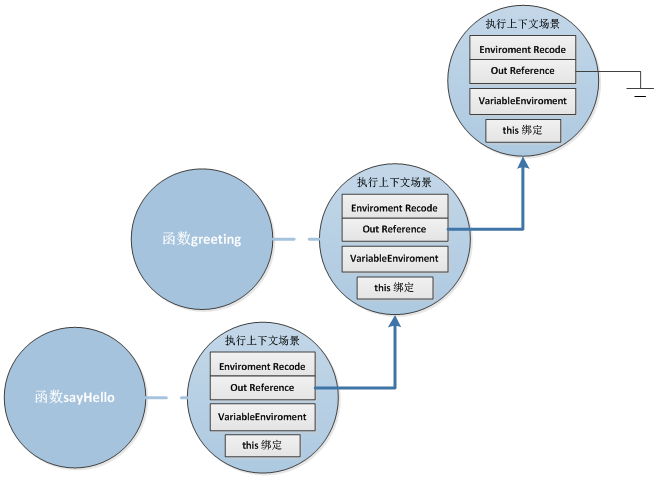http://coolshell.cn/articles/6731.html

#### 什么是闭包

• 闭包就是函数的局部变量集合，只是这些局部变量在函数返回后会继续存在。
• 闭包就是就是函数的“堆栈”在函数返回后并不释放，我们也可以理解为这些函数堆栈并不在栈上分配而是在堆上分配
• 当在一个函数内定义另外一个函数就会产生闭包

 1234567 `function` `greeting(name) {``    ``var` `text = ``'Hello '` `+ name; ``// local variable``    ``// 每次调用时，产生闭包，并返回内部函数对象给调用者``    ``return` `function``() { alert(text); }``}``var` `sayHello=greeting(``"Closure"``);``sayHello()  ``// 通过闭包访问到了局部变量text`

#### ECMAScript闭包模型

ECMAScript到底是如何实现闭包的呢？想深入了解的亲们可以获取ECMAScript 规范进行研究，我这里也只做一个简单的讲解，内容也是来自于网络。

• 文法环境（The LexicalEnvironment）
• 变量环境（The VariableEnvironment）
• this绑定#### 闭包的样列

 12345678910 `function` `say667() {``    ``// Local variable that ends up within closure``    ``var` `num = 666;``    ``var` `sayAlert = ``function``() { alert(num); }``    ``num++;``    ``return` `sayAlert;``}` `var` `sayAlert = say667();``sayAlert()`

 1234567891011121314 `function` `setupSomeGlobals() {``    ``// Local variable that ends up within closure``    ``var` `num = 666;``    ``// Store some references to functions as global variables``    ``gAlertNumber = ``function``() { alert(num); }``    ``gIncreaseNumber = ``function``() { num++; }``    ``gSetNumber = ``function``(x) { num = x; }``}``setupSomeGlobals(); ``// 为三个全局变量赋值``gAlertNumber(); ``//666``gIncreaseNumber();``gAlertNumber(); ``// 667``gSetNumber(12);``//``gAlertNumber();``//12`

 12345678910111213141516 `function` `buildList(list) {``    ``var` `result = [];``    ``for` `(``var` `i = 0; i < list.length; i++) {``        ``var` `item = ``'item'` `+ list[i];``        ``result.push( ``function``() {alert(item + ``' '` `+ list[i])} );``    ``}``    ``return` `result;``}` `function` `testList() {``    ``var` `fnlist = buildList([1,2,3]);``    ``// using j only to help prevent confusion - could use i``    ``for` `(``var` `j = 0; j < fnlist.length; j++) {``        ``fnlist[j]();``    ``}``}`

testList的执行结果是弹出item3 undefined窗口三次，因为这三个函数绑定了同一个闭包，而且item的值为最后计算的结果，但是当i跳出循环时i值为4，所以list的结果为undefined.

 12345678 `function` `sayAlice() {``    ``var` `sayAlert = ``function``() { alert(alice); }``    ``// Local variable that ends up within closure``    ``var` `alice = ``'Hello Alice'``;``    ``return` `sayAlert;``}``var` `helloAlice=sayAlice();``helloAlice();`

 123456789101112131415161718 `function` `newClosure(someNum, someRef) {``    ``// Local variables that end up within closure``    ``var` `num = someNum;``    ``var` `anArray = [1,2,3];``    ``var` `ref = someRef;``    ``return` `function``(x) {``        ``num += x;``        ``anArray.push(num);``        ``alert(``'num: '` `+ num +``        ``'\nanArray '` `+ anArray.toString() +``        ``'\nref.someVar '` `+ ref.someVar);``    ``}``}``closure1=newClosure(40,{someVar:``'closure 1'``});``closure2=newClosure(1000,{someVar:``'closure 2'``});` `closure1(5); ``// num:45 anArray[1,2,3,45] ref:'someVar closure1'``closure2(-10);``// num:990 anArray[1,2,3,990] ref:'someVar closure2'`

#### 闭包的应用

Singleton 单件：

 123456789101112131415 `var` `singleton = ``function` `() {``    ``var` `privateVariable;``    ``function` `privateFunction(x) {``        ``...privateVariable...``    ``}` `    ``return` `{``        ``firstMethod: ``function` `(a, b) {``            ``...privateVariable...``        ``},``        ``secondMethod: ``function` `(c) {``            ``...privateFunction()...``        ``}``    ``};``}();`

posted on 2012-03-09 11:39 SIMONE 阅读(297) 评论(0)  编辑  收藏 所属分类: JavaScript只有注册用户登录后才能发表评论。 网站导航: 相关文章: